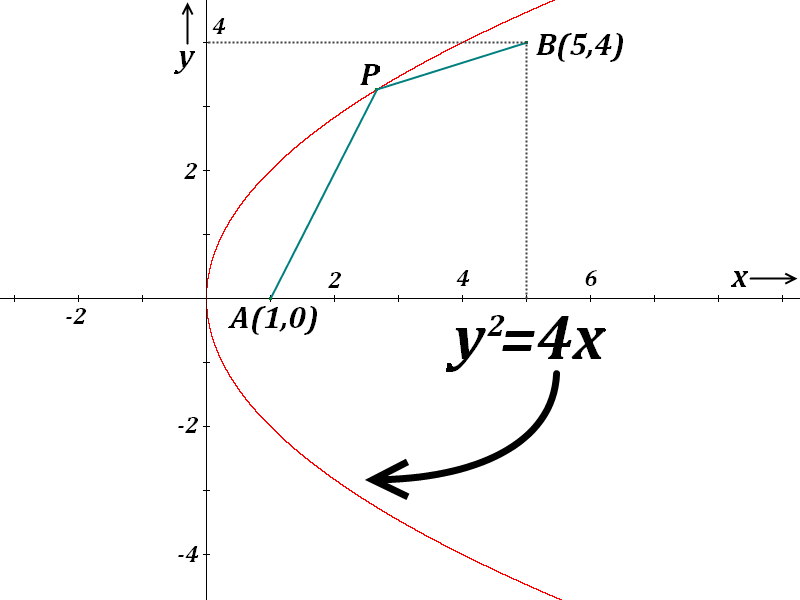# Parabola

Geometry Level 3Consider two points A (1,0), and B (5,4). Point P (whose coordinate is unknown yet) lies somewhere on parabola ${ y }^{ 2 }=4x$. As point P moves along the parabola, evaluate the minimum possible value of $\overline { PA } +\overline { PB }$.

×